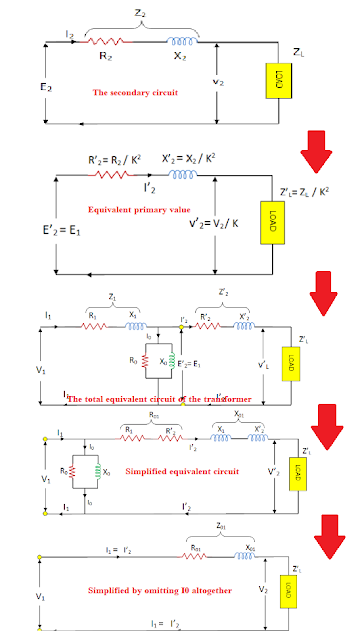WAZIPOINT Engineering Science & Technology: Transformer Equivalent Circuit Diagram

## How to draw the Equivalent Circuit Diagram of a Transformer?

If you want to draw the Transformer equivalent circuit, it would be like the below:Fig: Equivalent Circuit Diagram of a Transformer.

### What should be considered during drawing the Transformer equivalent circuit diagram?

Considering the two major parts of a transformer- primary winding and secondary winding with their parameters the equivalent circuit would be as above;

where,
R1 = Primary Winding Resistance.
R2= Secondary winding Resistance.
Iµ = Magnetizing Component,
Iw = Working Component,
This Iµ & Iw are connected in parallel across the primary circuit.

The value of E1 ( Primary e.m.f ) is obtained by subtracting vectorially I1Z1 from V1

The value of X0 = E1 / I0 and R0 = E1 /Iw

We know that the relation of E1 and E2 is E2 /E1 = N2 /N1 = K.  The K is known as the Transformation Ratio.

### Calculation of Transformer Equivalent Circuit Diagram

Now, you can easily calculate the total impedance to transfer voltage, current, and impedance either to the primary or the secondary from the equivalent circuit of a transformer  considering the parameters below:### Why Transformers Are Rated In KVA instate of KW?

From the below transformer open circuit or no-load test and short circuit or Impedance test on Transformer, it can be seen that Cu (Copper) loss of a transformer depends on current, and iron loss depends on voltage. This means the total transformer loss depends on Voltage and Current (VA). It does not depend on the phase angle between voltage and current. We can say the transformer loss is independent of the load power factor. This is the reason that transformers are rated in kVA instates of KW.